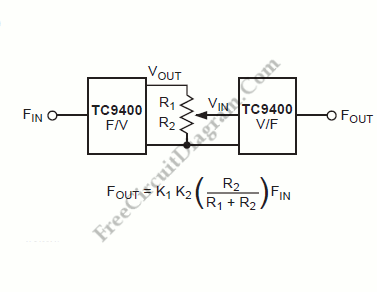# Infinite Resolution Frequency Multiplier/DividerNormally, frequency multiplier or divider has only discrete ratio operation since it uses digital divider as the main process for division or multiplication. By first converting the incoming frequency into a proportional DC voltage, we can perform frequency scaling easily with no restriction of discrete ratio operation. Here is the block diagram the scheme:We can accomplished it by using the TC9400 in the F/V mode. It is easy to scale this voltage up or down by use of a single potentiometer once the frequency is in a voltage format. Then, we can apply the resultant voltage to a TC9400 V/F converter, which generates a proportional output frequency. The division/multiplication factor can be any number, including fractions (K1 is simply Vout/Fin, while K2 is Fout/Vin) since the potentiometer is infinitely variable. [System’s block diagram source: Microchip Application Note]